Request a Free Counselling Session from our Expert Mentor# NCERT Solution for Class 12 Math Chapter 5

Swastik Classes’ NCERT Solution for Class 12 Mathematics Chapter 5, “Continuity and Differentiability,” is an essential study material designed to help students understand the concepts of continuity and differentiability in calculus. The chapter covers various topics, including continuity, differentiability, mean value theorem, and Rolle’s theorem. Swastik Classes, a leading coaching institute, has developed comprehensive NCERT solutions that provide step-by-step explanations and solved examples to help students develop a deeper understanding of the subject. These solutions are designed according to the latest CBSE syllabus, making them useful for students preparing for board exams or competitive exams like JEE and NEET. With the help of Swastik Classes’ NCERT solutions, students can improve their problem-solving skills and gain the confidence to tackle complex calculus problems. Overall, Swastik Classes’ NCERT Solution for Class 12 Mathematics Chapter 5 is an essential resource for students who want to excel in mathematics and build a strong foundation in calculus.

## Answers of Physics NCERT solutions for class 12 Chapter 5 Continuity & Differentiability

Chapter 5

Continuity & Differentiability

Exercise-5.1

Question 1:

Prove that the function is continuous at at and

Therefore, f is continuous at x = 0

Therefore, is continuous at x = −3

Therefore, f is continuous at x = 5

Question 2:

Examine the continuity of the function at

Thus, f is continuous at x = 3

Question 3:

Examine the following functions for continuity.

(a) (b)

(c)   (d)

(a) The given function is

It is evident that f is defined at every real number k and its value at k is k − 5.

It is also observed that,

Hence, f is continuous at every real number and therefore, it is a continuous function.

(b) The given function is

For any real number k ≠ 5, we obtain

Hence, f is continuous at every point in the domain of f and therefore, it is a continuous function.

(c) The given function is

For any real number c ≠ −5, we obtain

Hence, f is continuous at every point in the domain of f and therefore, it is a continuous function.

(d) The given function is

This function f is defined at all points of the real line.

Let c be a point on a real line. Then, c < 5 or c = 5 or c > 5

Case I: c < 5

Then, (c) = 5 − c

Therefore, f is continuous at all real numbers less than 5.

Case II : c = 5

Then,

Therefore, is continuous at x = 5

Case III: c > 5

Therefore, f is continuous at all real numbers greater than 5.

Hence, f is continuous at every real number and therefore, it is a continuous function.

Question 4:

Prove that the function  is continuous at x = n, where n is a positive integer.

The given function is fx=xn

It is evident that f is defined at all positive integers, n, and its value at n is nn.

Therefore, is continuous at n, where n is a positive integer.

Question 5:

Is the function f defined by

continuous at x = 0? At x = 1? At x = 2?

The given function f is

At x = 0,

It is evident that f is defined at 0 and its value at 0 is 0.

Therefore, f is continuous at x = 0

At x = 1,

is defined at 1 and its value at 1 is 1.

The left hand limit of f at x = 1 is,

The right hand limit of at x = 1 is,

Therefore, f is not continuous at x = 1

At = 2,

is defined at 2 and its value at 2 is 5.

Therefore, f is continuous at = 2

Question 6:

Find all points of discontinuity of f, where f is defined by

The given function f is

It is evident that the given function f is defined at all the points of the real line.

Let c be a point on the real line. Then, three cases arise.

(i)  c < 2

(ii)  c > 2

(iii) c = 2

Case (i) c < 2

Therefore, f is continuous at all points x, such that x < 2

Case (ii) c > 2

Therefore, f is continuous at all points x, such that x > 2

Case (iii) c = 2

Then, the left hand limit of at x = 2 is,

The right hand limit of f at x = 2 is,

It is observed that the left and right hand limit of f at x = 2 do not coincide.

Therefore, f is not continuous at x = 2

Hence, x = 2 is the only point of discontinuity of f.

Question 7:

Find all points of discontinuity of f, where f is defined by

The given function f is

The given function f is defined at all the points of the real line.

Let c be a point on the real line.

Case I:

Therefore, f is continuous at all points x, such that x < −3

Case II:

Therefore, f is continuous at x = −3

Case III:

Therefore, f is continuous in (−3, 3).

Case IV:

If c = 3, then the left hand limit of at x = 3 is,

The right hand limit of at x = 3 is,

It is observed that the left and right hand limit of f at x = 3 do not coincide.

Therefore, f is not continuous at x = 3

Case V:

Therefore, f is continuous at all points x, such that x > 3

Hence, x = 3 is the only point of discontinuity of f.

Question 8:

Find all points of discontinuity of f, where f is defined by

The given function f is

It is known that,

Therefore, the given function can be rewritten as

The given function f is defined at all the points of the real line.

Let c be a point on the real line.

Case I:

Therefore, f is continuous at all points x < 0

Case II:

If c = 0, then the left hand limit of at x = 0 is,

The right hand limit of at x = 0 is,

It is observed that the left and right hand limit of f at x = 0 do not coincide.

Therefore, f is not continuous at x = 0

Case III:

Therefore, f is continuous at all points x, such that x > 0

Hence, x = 0 is the only point of discontinuity of f.

Question 9:

Find all points of discontinuity of f, where f is defined by

The given function f is

It is known that,

Therefore, the given function can be rewritten as

Let c be any real number. Then,

Also,

Therefore, the given function is a continuous function.

Hence, the given function has no point of discontinuity.

Question 10:

Find all points of discontinuity of f, where f is defined by

The given function f is

The given function f is defined at all the points of the real line.

Let c be a point on the real line.

Case I:

Therefore, f is continuous at all points x, such that x < 1

Case II:

The left hand limit of at x = 1 is,

The right hand limit of at x = 1 is,

Therefore, f is continuous at x = 1

Case III:

Therefore, f is continuous at all points x, such that x > 1

Hence, the given function has no point of discontinuity.

Question 11:

Find all points of discontinuity of f, where f is defined by

The given function f is

The given function f is defined at all the points of the real line.

Let c be a point on the real line.

Case I:

Therefore, f is continuous at all points x, such that x < 2

Case II:

Therefore, f is continuous at x = 2

Case III:

Therefore, f is continuous at all points x, such that x > 2

Thus, the given function f is continuous at every point on the real line.

Hence, has no point of discontinuity.

Question 12:

Find all points of discontinuity of f, where f is defined by

The given function f is

The given function f is defined at all the points of the real line.

Let c be a point on the real line.

Case I:

Therefore, f is continuous at all points x, such that x < 1

Case II:

If c = 1, then the left hand limit of f at x = 1 is,

The right hand limit of f at = 1 is,

It is observed that the left and right hand limit of f at x = 1 do not coincide.

Therefore, f is not continuous at x = 1

Case III:

Therefore, f is continuous at all points x, such that x > 1

Thus, from the above observation, it can be concluded that x = 1 is the only point of discontinuity of f.

Question 13:

Is the function defined by

a continuous function?

The given function is

The given function f is defined at all the points of the real line.

Let c be a point on the real line.

Case I:

Therefore, f is continuous at all points x, such that x < 1

Case II:

The left hand limit of at x = 1 is,

The right hand limit of f at = 1 is,

It is observed that the left and right hand limit of f at x = 1 do not coincide.

Therefore, f is not continuous at x = 1

Case III:

Therefore, f is continuous at all points x, such that x > 1

Thus, from the above observation, it can be concluded that x = 1 is the only point of discontinuity of f.

Question 14:

Discuss the continuity of the function f, where f is defined by

The given function is

The given function is defined at all points of the interval [0, 10].

Let c be a point in the interval [0, 10].

Case I:

Therefore, f is continuous in the interval [0, 1).

Case II:

The left hand limit of at x = 1 is,

The right hand limit of f at = 1 is,

It is observed that the left and right hand limits of f at x = 1 do not coincide.

Therefore, f is not continuous at x = 1

Case III:

Therefore, f is continuous at all points of the interval (1, 3).

Case IV:

The left hand limit of at x = 3 is,

The right hand limit of f at = 3 is,

It is observed that the left and right hand limits of f at x = 3 do not coincide.

Therefore, f is not continuous at x = 3

Case V:

Therefore, f is continuous at all points of the interval (3, 10].

Hence, is not continuous at = 1 and = 3

Question 15:

Discuss the continuity of the function f, where f is defined by

The given function is

The given function is defined at all points of the real line.

Let c be a point on the real line.

Case I:

Therefore, f is continuous at all points x, such that x < 0

Case II:

The left hand limit of at x = 0 is,

The right hand limit of f at = 0 is,

Therefore, f is continuous at x = 0

Case III:

Therefore, f is continuous at all points of the interval (0, 1).

Case IV:

The left hand limit of at x = 1 is,

The right hand limit of f at = 1 is,

It is observed that the left and right hand limits of f at x = 1 do not coincide.

Therefore, f is not continuous at x = 1

Case V:

Therefore, f is continuous at all points x, such that x > 1

Hence, is not continuous only at = 1

Question 16:

Discuss the continuity of the function f, where f is defined by

The given function f is

The given function is defined at all points of the real line.

Let c be a point on the real line.

Case I:

Therefore, f is continuous at all points x, such that x < −1

Case II:

The left hand limit of at x = −1 is,

The right hand limit of f at = −1 is,

Therefore, f is continuous at x = −1

Case III:

Therefore, f is continuous at all points of the interval (−1, 1).

Case IV:

The left hand limit of at x = 1 is,

The right hand limit of f at = 1 is,

Therefore, f is continuous at x = 2

Case V:

Therefore, f is continuous at all points x, such that x > 1

Thus, from the above observations, it can be concluded that f is continuous at all points of the real line.

Question 17:

Find the relationship between a and b so that the function f defined by

is continuous at = 3.

The given function f is

If f is continuous at x = 3, then

Therefore, from (1), we obtain

Therefore, the required relationship is given by,

*Question 18:

For what value of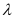is the function defined by

continuous at x = 0? What about continuity at x = 1?

The given function f is

If f is continuous at x = 0, then

Therefore, there is no value of λ for which f is continuous at x = 0

At x = 1,

f (1) = 4x + 1 = 4 × 1 + 1 = 5

Therefore, for any values of λ, f is continuous at x = 1

Question 19:

Show that the function defined by  is discontinuous at all integral point. Here  denotes the greatest integer less than or equal to x.

The given function is

It is evident that g is defined at all integral points.

Let n be an integer.

Then,

The left hand limit of at x = n is,

The right hand limit of f at n is,

It is observed that the left and right hand limits of f at x = n do not coincide.

Therefore, f is not continuous at x = n

Hence, g is discontinuous at all integral points.

Question 20:

Is the function defined by  continuous at =π?

The given function is

It is evident that f is defined at =π.

Therefore, the given function f is continuous at = π

Question 21:

Discuss the continuity of the following functions.

(a)  f (x) = sin x + cos x

(b)  f (x) = sin x − cos x

(c)  f (x) = sin x × cos x

It is known that if and are two continuous functions, then and g.h are also continuous.

It has to proved first that g (x) = sin and h (x) = cos x are continuous functions.

Let (x) = sin x

It is evident that g (x) = sin x is defined for every real number.

Let be a real number. Put x = c + h

If x → c, then h → 0

Therefore, g is a continuous function.

Let h (x) = cos x

It is evident that h (x) = cos x is defined for every real number.

Let be a real number. Put x = c + h

If x → c, then h → 0

(c) = cos c

Therefore, h is a continuous function.

Therefore, it can be concluded that

(a)  f (x) = g (x) + h (x) = sin x + cos x is a continuous function

(b)  f (x) = g (x) − h (x) = sin x − cos x is a continuous function

(c)  f (x) = g (x) × h (x) = sin x × cos x is a continuous function

Question 22:

Discuss the continuity of the cosine, cosecant, secant and cotangent functions,

It is known that if and are two continuous functions, then

It has to be proved first that g (x) = sin and h (x) = cos x are continuous functions.

Let (x) = sin x

It is evident that g (x) = sin x is defined for every real number.

Let be a real number. Put x = c + h

If x c, then h → 0

Therefore, g is a continuous function.

Let h (x) = cos x

It is evident that h (x) = cos x is defined for every real number.

Let be a real number. Put x = c + h

If x → c, then h → 0

(c) = cos c

Therefore, h (x) = cos x is continuous function.

It can be concluded that,

Therefore, cosecant is continuous except at np, n ε Z

Therefore, secant is continuous except at

Therefore, cotangent is continuous except at np, n ε Z

*Question 23:

Find the points of discontinuity of f, where

The given function f is

It is evident that f is defined at all points of the real line.

Let c be a real number.

Case I:

Therefore, f is continuous at all points x, such that x < 0

Case II:

Therefore, f is continuous at all points x, such that x > 0

Case III:

The left hand limit of f at x = 0 is,

The right hand limit of f at x = 0 is,

Therefore, f is continuous at x = 0

From the above observations, it can be concluded that f is continuous at all points of the real line.

Thus, f has no point of discontinuity.

*Question 24:

Determine if f defined by

is a continuous function?

The given function f is

It is evident that f is defined at all points of the real line.

Let c be a real number.

Case I:

Therefore, f is continuous at all points ≠ 0

Case II:

Therefore, f is continuous at x = 0

From the above observations, it can be concluded that f is continuous at every point of the real line.

Thus, f is a continuous function.

Question 25:

Examine the continuity of f, where f is defined by

The given function f is

It is evident that f is defined at all points of the real line.

Let c be a real number.

Case I:

Therefore, f is continuous at all points x, such that x ≠ 0

Case II:

Therefore, f is continuous at x = 0

From the above observations, it can be concluded that f is continuous at every point of the real line.

Thus, f is a continuous function.

*Question 26:

Find the values of so that the function f is continuous at the indicated point.

The given function f is

The given function f is continuous at , if f is defined at  and if the value of the f at  equals the limit of f at

It is evident that is defined at  and

Therefore, the required value of k is 6.

Question 27:

Find the values of so that the function f is continuous at the indicated point.

The given function is

The given function f is continuous at x = 2, if f is defined at x = 2 and if the value of f at x = 2 equals the limit of f at x = 2

It is evident that is defined at x = 2 and

Therefore, the required value of k is

Question 28:

Find the values of so that the function f is continuous at the indicated point.

The given function is

The given function f is continuous at x = p, if f is defined at x = p and if the value of f at x = p equals the limit of f at x = p

It is evident that is defined at x = p and

Therefore, the required value of k is

Question 29:

Find the values of so that the function f is continuous at the indicated point.

The given function is

The given function f is continuous at x = 5, if f is defined at x = 5 and if the value of f at x = 5 equals the limit of f at x = 5

It is evident that is defined at x = 5 and

Therefore, the required value of k is

Question 30:

Find the values of a and b such that the function defined by

is a continuous function.

The given function is

It is evident that the given function f is defined at all points of the real line.

If f is a continuous function, then f is continuous at all real numbers.

In particular, f is continuous at = 2 and = 10

Since f is continuous at = 2, we obtain

Since f is continuous at = 10, we obtain

On subtracting equation (1) from equation (2), we obtain

8a = 16

⇒ a = 2

By putting a = 2 in equation (1), we obtain

2 × 2 + b = 5

⇒ 4 + b = 5

⇒ b = 1

Therefore, the values of a and b for which f is a continuous function are 2 and 1 respectively.

Question 31:

Show that the function defined by f (x) = cos (x2) is a continuous function.

The given function is (x) = cos (x2)

This function f is defined for every real number and f can be written as the composition of two functions as,

f = g o h, where g (x) = cos x and h (x) = x2

It has to be first proved that (x) = cos x and h (x) = x2 are continuous functions.

It is evident that g is defined for every real number.

Let c be a real number.

Then, g (c) = cos c

Therefore, g (x) = cos x is continuous function.

h (x) = x2

Clearly, h is defined for every real number.

Let k be a real number, then h (k) = k2

Therefore, h is a continuous function.

It is known that for real valued functions and h, such that (h) is defined at c, if is continuous at and if is continuous at (c), then (g) is continuous at c.

Therefore,  is a continuous function.

Question 32:

Show that the function defined by  is a continuous function.

The given function is

This function f is defined for every real number and f can be written as the composition of two functions as,

f = g o h, where and

It has to be first proved that  and  are continuous functions.

Clearly, g is defined for all real numbers.

Let c be a real number.

Case I:

Therefore, g is continuous at all points x, such that x < 0

Case II:

Therefore, g is continuous at all points x, such that x > 0

Case III:

Therefore, g is continuous at x = 0

From the above three observations, it can be concluded that g is continuous at all points.

(x) = cos x

It is evident that h (x) = cos x is defined for every real number.

Let be a real number. Put x = c + h

If x → c, then h → 0

(c) = cos c

Therefore, h (x) = cos x is a continuous function.

It is known that for real valued functions and h,such that (h) is defined at c, if is continuous at and if is continuous at (c), then (g) is continuous at c.

Therefore,  is a continuous function.

Question 33:

Examine that  is a continuous function.

This function f is defined for every real number and f can be written as the composition of two functions as,

f = g o h, where and

It has to be proved first that  and  are continuous functions.

Clearly, g is defined for all real numbers.

Let c be a real number.

Case I:

Therefore, g is continuous at all points x, such that x < 0

Case II:

Therefore, g is continuous at all points x, such that x > 0

Case III:

Therefore, g is continuous at x = 0

From the above three observations, it can be concluded that g is continuous at all points.

(x) = sin x

It is evident that h (x) = sin x is defined for every real number.

Let be a real number. Put x = c + k

If x → c, then k → 0

(c) = sin c

Therefore, h is a continuous function.

It is known that for real valued functions and h,such that (h) is defined at c, if is continuous at and if is continuous at (c), then (g) is continuous at c.

Therefore,  is a continuous function.

Question 34:

Find all the points of discontinuity of defined by

The given function is

The two functions, g and h, are defined as

Then, f = − h

The continuity of g and is examined first.

Clearly, g is defined for all real numbers.

Let c be a real number.

Case I:

Therefore, g is continuous at all points x, such that x < 0

Case II:

Therefore, g is continuous at all points x, such that x > 0

Case III:

Therefore, g is continuous at x = 0

From the above three observations, it can be concluded that g is continuous at all points.

Clearly, h is defined for every real number.

Let be a real number.

Case I:

Therefore, h is continuous at all points x, such that x < −1

Case II:

Therefore, h is continuous at all points x, such that x > −1

Case III:

Therefore, h is continuous at x = −1

From the above three observations, it can be concluded that h is continuous at all points of the real line.

g and h are continuous functions. Therefore, g − is also a continuous function.

Therefore, has no point of discontinuity.

Exercise-3.2

Question 1:

Differentiate the functions with respect to x.

Let fx=sin x2+5 , u(x)=x2+5, and v(t)=sint

Alternate method

Question 2:

Differentiate the functions with respect to x.

Thus, is a composite function of two functions.

Put t = u (x) = sin x

By chain rule,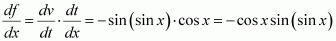Alternate method

Question 3:

Differentiate the functions with respect to x.

Thus, is a composite function of two functions, u and v.

Put t = u (x) = ax + b

Hence, by chain rule, we obtain

Alternate method

Question 4:

Differentiate the functions with respect to x.

Thus, is a composite function of three functions, u, v, and w.

Hence, by chain rule, we obtain

Alternate method

Question 5:

Differentiate the functions with respect to x.

The given function is where g (x) = sin (ax + b) and

h (x) = cos (cx d)

∴ is a composite function of two functions, u and v.

Therefore, by chain rule, we obtain

h is a composite function of two functions, p and q.

Put y = p (x) = cx d

Therefore, by chain rule, we obtain

Question 6:

Differentiate the functions with respect to x.

The given function is

Question 7:

Differentiate the functions with respect to x.

Question 8:

Differentiate the functions with respect to x.

Clearly, is a composite function of two functions, and v, such that

By using chain rule, we obtain

Alternate method

*Question 9:

Prove that the function given by  is not differentiable at x = 1.

The given function is

It is known that a function f is differentiable at a point x = c in its domain if both

and are finite and equal.

To check the differentiability of the given function at x = 1,

consider the left hand limit of f at x = 1

Since the left and right hand limits of f at x = 1 are not equal, f is not differentiable at x = 1

*Question 10:

Prove that the greatest integer function defined by is not

differentiable at x = 1 and x = 2.

The given function f is

It is known that a function f is differentiable at a point x = c in its domain if both and are finite and equal.

To check the differentiability of the given function at x = 1, consider the left hand limit of f at x = 1

Since the left and right hand limits of f at x = 1 are not equal, f is not differentiable at

x = 1

To check the differentiability of the given function at x = 2, consider the left hand limit

of f at x = 2

Since the left and right hand limits of f at x = 2 are not equal, f is not differentiable at x = 2

Exercise-3.2

Question 1:

Find

The given relationship is

Differentiating this relationship with respect to x, we obtain

Question 2:

Find

The given relationship is

Differentiating this relationship with respect to x, we obtain

Question 3:

Find

The given relationship is

Differentiating this relationship with respect to x, we obtain

Using chain rule, we obtain

and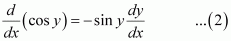From (1) and (2), we obtain

Question 4:

Find

The given relationship is

Differentiating this relationship with respect to x, we obtain

Question 5:

Find

The given relationship is

Differentiating this relationship with respect to x, we obtain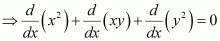[Derivative of constant function is 0]

Question 6:

Find

The given relationship is

Differentiating this relationship with respect to x, we obtain

*Question 7:

Find

The given relationship is

Differentiating this relationship with respect to x, we obtain

Using chain rule, we obtain

From (1), (2), and (3), we obtain

Question 8:

Find

The given relationship is

Differentiating this relationship with respect to x, we obtain

Question 9: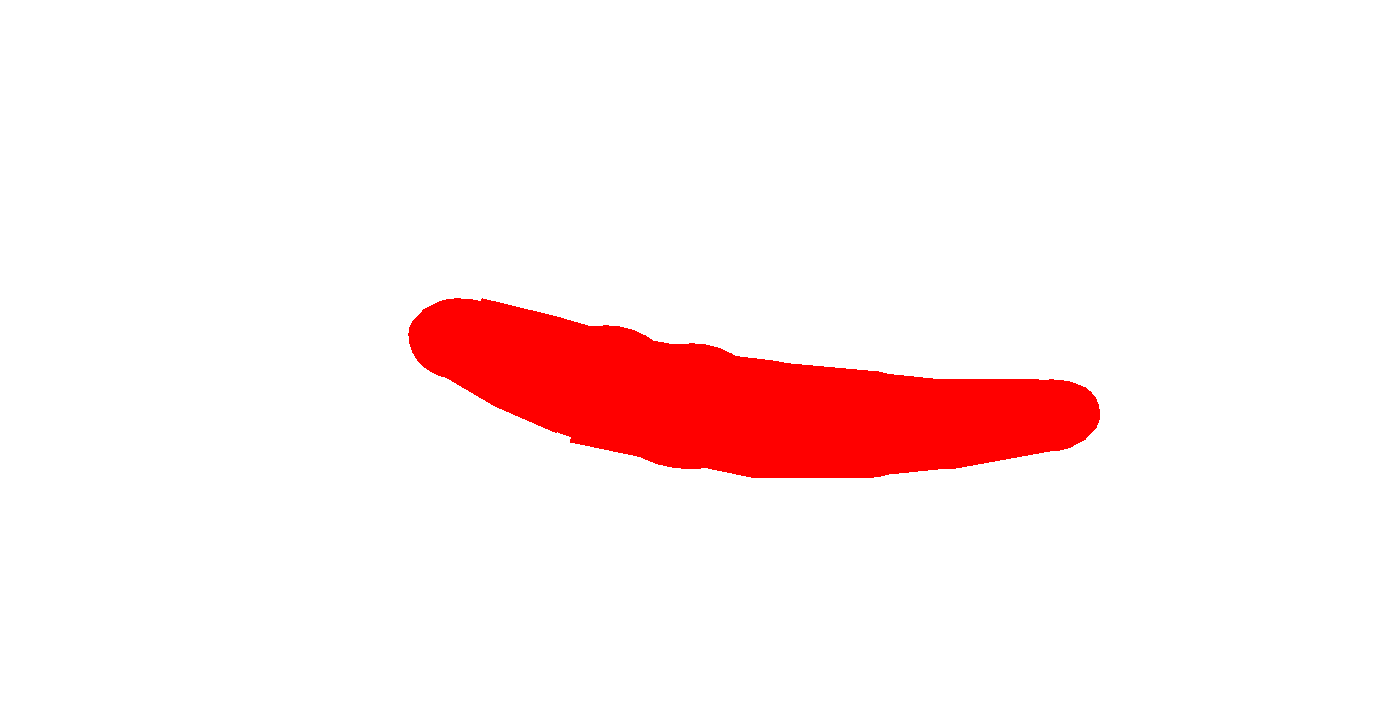Find

Answer:We have

Put

We have,y = sin-12×1 + x2put x = tan θ ⇒ θ = tan-1xNow,    y = sin-12 tan θ1 + tan2θ⇒y = sin-1sin 2θ, as sin 2θ=2 tan θ1 + tan2θ⇒y = 2θ,  as sin-1sin x = x ⇒ y = 2 tan-1x⇒dydx = 2 × 11 + x2, because dtan-1xdx=11 + x2 ⇒ dy dx = 21 + x2

Question 10:

Find

The given relationship is

It is known that,

Comparing equations (1) and (2), we obtain

Differentiating this relationship with respect to x, we obtain

Question 11:

Find

The given relationship is,

On comparing L.H.S. and R.H.S. of the above relationship, we obtain

Differentiating this relationship with respect to x, we obtain

*Question 12:

Find

The given relationship is

Differentiating this relationship with respect to x, we obtain

Using chain rule, we obtain

From (1), (2), and (3), we obtain

Alternate method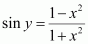Differentiating this relationship with respect to x, we obtain

Question 13:

Find

The given relationship is

Differentiating this relationship with respect to x, we obtain

*Question 14:

Find

The given relationship is

Differentiating this relationship with respect to x, we obtain

Question 15:

Find

The given relationship is

Differentiating this relationship with respect to x, we obtain

Exercise-5.4

Question 1:

Differentiate the following w.r.t. x:

Let

By using the quotient rule, we obtain

Question 2:

Differentiate the following w.r.t. x:

Let

By using the chain rule, we obtain

Question 3:

Differentiate the following w.r.t. x:

Let

By using the chain rule, we obtain

*Question 4:

Differentiate the following w.r.t. x:

Let

By using the chain rule, we obtain

Question 5:

Differentiate the following w.r.t. x:

Let

By using the chain rule, we obtain

*Question 6:

Differentiate the following w.r.t. x:

Question 7:

Differentiate the following w.r.t. x:

Let

Then,

By differentiating this relationship with respect to x, we obtain

Question 8:

Differentiate the following w.r.t. x:

Let

By using the chain rule, we obtain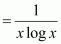x > 1

*Question 9:

Differentiate the following w.r.t. x:

Let

By using the quotient rule, we obtain

*Question 10:

Differentiate the following w.r.t. x:

Let

By using the chain rule, we obtain

Exercise-5.5

Question 1:

Differentiate the function with respect to x.

Taking logarithm on both the sides, we obtain

Differentiating both sides with respect to x, we obtain

Question 2:

Differentiate the function with respect to x.

Taking logarithm on both the sides, we obtain

Differentiating both sides with respect to x, we obtain

Question 3:

Differentiate the function with respect to x.

Taking logarithm on both the sides, we obtain

Differentiating both sides with respect to x, we obtain

Question 4:

Differentiate the function with respect to x.

xx

Taking logarithm on both the sides, we obtain

Differentiating both sides with respect to x, we obtain

v = 2sin x

Taking logarithm on both the sides with respect to x, we obtain

Differentiating both sides with respect to x, we obtain

Question 5:

Differentiate the function with respect to x.

Taking logarithm on both the sides, we obtain

Differentiating both sides with respect to x, we obtain

Question 6:

Differentiate the function with respect to x.

Differentiating both sides with respect to x, we obtain

Differentiating both sides with respect to x, we obtain

Therefore, from (1), (2), and (3), we obtain

Question 7:

Differentiate the function with respect to x.

= (log x)x

Differentiating both sides with respect to x, we obtain

Differentiating both sides with respect to x, we obtain

Therefore, from (1), (2), and (3), we obtain

*Question 8:

Differentiate the function with respect to x.

Differentiating both sides with respect to x, we obtain

Therefore, from (1), (2), and (3), we obtain

*Question 9:

Differentiate the function with respect to x.

Differentiating both sides with respect to x, we obtain

Differentiating both sides with respect to x, we obtain

From (1), (2), and (3), we obtain

*Question 10:

Differentiate the function with respect to x.

Differentiating both sides with respect to x, we obtain

Differentiating both sides with respect to x, we obtain

From (1), (2), and (3), we obtain

*Question 11:

Differentiate the function with respect to x.

Differentiating both sides with respect to x, we obtain

Differentiating both sides with respect to x, we obtain

From (1), (2), and (3), we obtain

Question 12:

Find  of function.

The given function is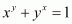Let xy = u and yx = v

Then, the function becomes u v = 1

Differentiating both sides with respect to x, we obtain

Differentiating both sides with respect to x, we obtain

From (1), (2), and (3), we obtain

*Question 13:

Find  of function.

The given function is

Taking logarithm on both the sides, we obtain

Differentiating both sides with respect to x, we obtain

Question 14:

Find  of function.

The given function is

Taking logarithm on both the sides, we obtain

Differentiating both sides, we obtain

Question 15:

Find  of function.

The given function is

Taking logarithm on both the sides, we obtain

Differentiating both sides with respect to x, we obtain

Question 16:

Find the derivative of the function given by  and hence find

The given relationship is

Taking logarithm on both the sides, we obtain

Differentiating both sides with respect to x, we obtain

Question 18:

If uv and w are functions of x, then show that

in two ways-first by repeated application of product rule, second by logarithmic differentiation.

Let

By applying product rule, we obtain

By taking logarithm on both sides of the equation , we obtain

Differentiating both sides with respect to x, we obtain

Exercise-5.6

Question 1:

If x and y are connected parametrically by the equation, without eliminating the parameter, find .

The given equations are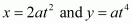Question 2:

If x and y are connected parametrically by the equation, without eliminating the parameter, find .

x = a cos θy = b cos θ

The given equations are x = a cos θ and y = b cos θ

*Question 3:

If x and y are connected parametrically by the equation, without eliminating the parameter, find .

x = sin ty = cos 2t

The given equations are x = sin t and y = cos 2t

Question 4:

If x and y are connected parametrically by the equation, without eliminating the parameter, find .

The given equations are and

Question 5:

If x and y are connected parametrically by the equation, without eliminating the parameter, find .

The given equations are

Question 6:

If x and y are connected parametrically by the equation, without eliminating the parameter, find .

The given equations are

Question 7:

If x and y are connected parametrically by the equation, without eliminating the parameter, find .

The given equations are

Question 8:

If x and y are connected parametrically by the equation, without eliminating the parameter, find .

The given equations are

Question 9:

If x and y are connected parametrically by the equation, without eliminating the parameter, find .

The given equations are

*Question 10:

If x and y are connected parametrically by the equation, without eliminating the parameter, find .

The given equations are

*Question 11:

If

The given equations are

Hence, proved.

Exercise-5.7

Question 1:

Find the second order derivatives of the function.

Let

Then,

Question 2:

Find the second order derivatives of the function.

Let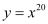Then,

Question 3:

Find the second order derivatives of the function.

Let

Then,

Question 4:

Find the second order derivatives of the function.

Let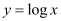Then,

Question 5:

Find the second order derivatives of the function.

Let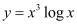Then,

Question 6:

Find the second order derivatives of the function.

Let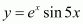Then,

Question 7:

Find the second order derivatives of the function.

Let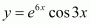Then,

Question 8:

Find the second order derivatives of the function.

Let

Then,

*Question 9:

Find the second order derivatives of the function.

Let

Then,

Question 10:

Find the second order derivatives of the function.

Let

Then,

*Question 11:

If , prove that

It is given that,

Then,

Hence, proved.

Question 12:

If  find in terms of y alone.

It is given that,

Then,

Question 13:

If show that

It is given that,

Then,

Hence, proved.

*Question 14:

If show that

It is given that,

Then,

Hence, proved.

Question 15:

If , show that

It is given that,

Then,

Hence, proved.

Question 16:

If show that

The given relationship is

Taking logarithm on both the sides, we obtain

Differentiating this relationship with respect to x, we obtain

Hence, proved.

Question 17:

If , show that

The given relationship is

Then,

Hence, proved.

Exercise-5.8

Question 1:

Verify Rolle’s Theorem for the function

The given function,, being a polynomial function, is continuous in [−4, 2] and is differentiable in (−4, 2).

∴ f (−4) = f (2) = 0

⇒ The value of f (x) at −4 and 2 coincides.

Rolle’s Theorem states that there is a point c ∈ (−4, 2) such that

Hence, Rolle’s Theorem is verified for the given function.

Question 2:

Examine if Rolle’s Theorem is applicable to any of the following functions. Can you say some thing about the converse of Rolle’s Theorem from these examples?

(i) for

(ii)  for

(iii) for

By Rolle’s Theorem, for a function , if

(a) f is continuous on [ab]

(b) f is differentiable on (ab)

(c)  (a) = f (b)

then, there exists some c ∈ (ab) such that

Therefore, Rolle’s Theorem is not applicable to those functions that do not satisfy any of the three conditions of the hypothesis.

(i)  for

It is evident that the given function f (x) is not continuous at every integral point.

In particular, f(x) is not continuous at = 5 and = 9

⇒ f (x) is not continuous in [5, 9].

The differentiability of f in (5, 9) is checked as follows.

Let be an integer such that n ∈ (5, 9).

Since the left and right hand limits of f at x = n are not equal, f is not differentiable at x = n

is not differentiable in (5, 9).

It is observed that f does not satisfy all the conditions of the hypothesis of Rolle’s Theorem.

Hence, Rolle’s Theorem is not applicable for for

(ii)  for

It is evident that the given function f (x) is not continuous at every integral point.

In particular, f(x) is not continuous at = −2 and = 2

⇒ f (x) is not continuous in [−2, 2].

The differentiability of f in (−2, 2) is checked as follows.

Let be an integer such that n ∈ (−2, 2).

Since the left and right hand limits of f at x = n are not equal, f is not differentiable at x = n

is not differentiable in (−2, 2).

It is observed that f does not satisfy all the conditions of the hypothesis of Rolle’s Theorem.

Hence, Rolle’s Theorem is not applicable for for .

(iii) for

It is evident that f, being a polynomial function, is continuous in [1, 2] and is differentiable in (1, 2).

(1) ≠ f (2)

It is observed that f does not satisfy a condition of the hypothesis of Rolle’s Theorem.

Hence, Rolle’s Theorem is not applicable for for

*Question 3:

If  is a differentiable function and if  does not vanish anywhere, then prove that

It is given that is a differentiable function.

Since every differentiable function is a continuous function, we obtain

(a) f is continuous on [−5, 5].

(b) is differentiable on (−5, 5).

Therefore, by the Mean Value Theorem, there exists c ∈ (−5, 5) such that

It is also given that  does not vanish anywhere.

Hence, proved.

Question 4:

Verify Mean Value Theorem, if   in the interval where and, b = 4

The given function is

f, being a polynomial function, is continuous in [1, 4] and is differentiable in (1, 4) whose derivative is 2x − 4.

Mean Value Theorem states that there is a point c ∈ (1, 4) such that

Hence, Mean Value Theorem is verified for the given function.

*Question 5:

Verify Mean Value Theorem, if  in the interval [ab], where a = 1 and b = 3. Find all  for which

The given function f is

f, being a polynomial function, is continuous in [1, 3] and is differentiable in (1, 3) whose derivative is 3x2 − 10x − 3.

Mean Value Theorem states that there exist a point c ∈ (1, 3) such thatHence, Mean Value Theorem is verified for the given function and  is the only point for which

Question 6:

Examine the applicability of Mean Value Theorem for all three functions given in the above exercise 2.

Mean Value Theorem states that for a function if

(a)  f is continuous on [ab]

(b) f is differentiable on (ab)

then, there exists some c ∈ (ab) such that

Therefore, Mean Value Theorem is not applicable to those functions that do not satisfy any of the two conditions of the hypothesis.

(i)

It is evident that the given function f (x) is not continuous at every integral point.

In particular, f(x) is not continuous at = 5 and = 9

⇒ f (x) is not continuous in [5, 9].

The differentiability of f in (5, 9) is checked as follows.

Let be an integer such that n ∈ (5, 9).

Since the left and right hand limits of f at x = n are not equal, f is not differentiable at x = n

is not differentiable in (5, 9).

It is observed that f does not satisfy all the conditions of the hypothesis of Mean Value Theorem.

Hence, Mean Value Theorem is not applicable for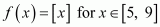.

(ii)

It is evident that the given function f (x) is not continuous at every integral point.

In particular, f(x) is not continuous at = −2 and = 2

⇒ f (x) is not continuous in [−2, 2].

The differentiability of f in (−2, 2) is checked as follows.

Let be an integer such that n ∈ (−2, 2).

Since the left and right hand limits of f at x = n are not equal, f is not differentiable at x = n

is not differentiable in (−2, 2).

It is observed that f does not satisfy all the conditions of the hypothesis of Mean Value Theorem.

Hence, Mean Value Theorem is not applicable for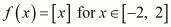.

(iii)

It is evident that f, being a polynomial function, is continuous in [1, 2] and is differentiable in (1, 2).

It is observed that f satisfies all the conditions of the hypothesis of Mean Value Theorem.

Hence, Mean Value Theorem is applicable for .

It can be proved as follows.

Miscellaneous exercise

Differentiate wrt x the function in question 1 to 11

Question 1:

Using chain rule, we obtain

Question 2:

Question 3:

Taking logarithm on both the sides, we obtain

Differentiating both sides with respect to x, we obtain

Question 4:

Using chain rule, we obtain

Question 5:

*Question 6:

Therefore, equation (1) becomes

*Question 7:

Taking logarithm on both the sides, we obtain

Differentiating both sides with respect to x, we obtain

Question 8:

for some constant a and b.

By using chain rule, we obtain

Question 9:

Taking logarithm on both the sides, we obtain

Differentiating both sides with respect to x, we obtain

Question 10:

for some fixed  and

Differentiating both sides with respect to x, we obtain

Differentiating both sides with respect to x, we obtain

s = aa

Since a is constant, aa is also a constant.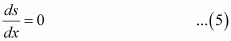From (1), (2), (3), (4), and (5), we obtain

Question 11: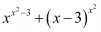, for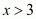Differentiating both sides with respect to x, we obtain

Differentiating with respect to x, we obtain

Also,

Differentiating both sides with respect to x, we obtain

Substituting the expressions of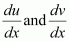in equation (1), we obtain

Question 12:

Find if

*Question 13:

Find if

*Question 14:

If for, −1 < x <1, prove that

It is given that,

Differentiating both sides with respect to x, we obtain

Hence, proved.

*Question 15:

If , for some  prove that

is a constant independent of a and b.

It is given that,

Differentiating both sides with respect to x, we obtain

Hence, proved.

Question 16:

If  with  prove that

Then, equation (1) reduces to

Hence, proved.

*Question 17: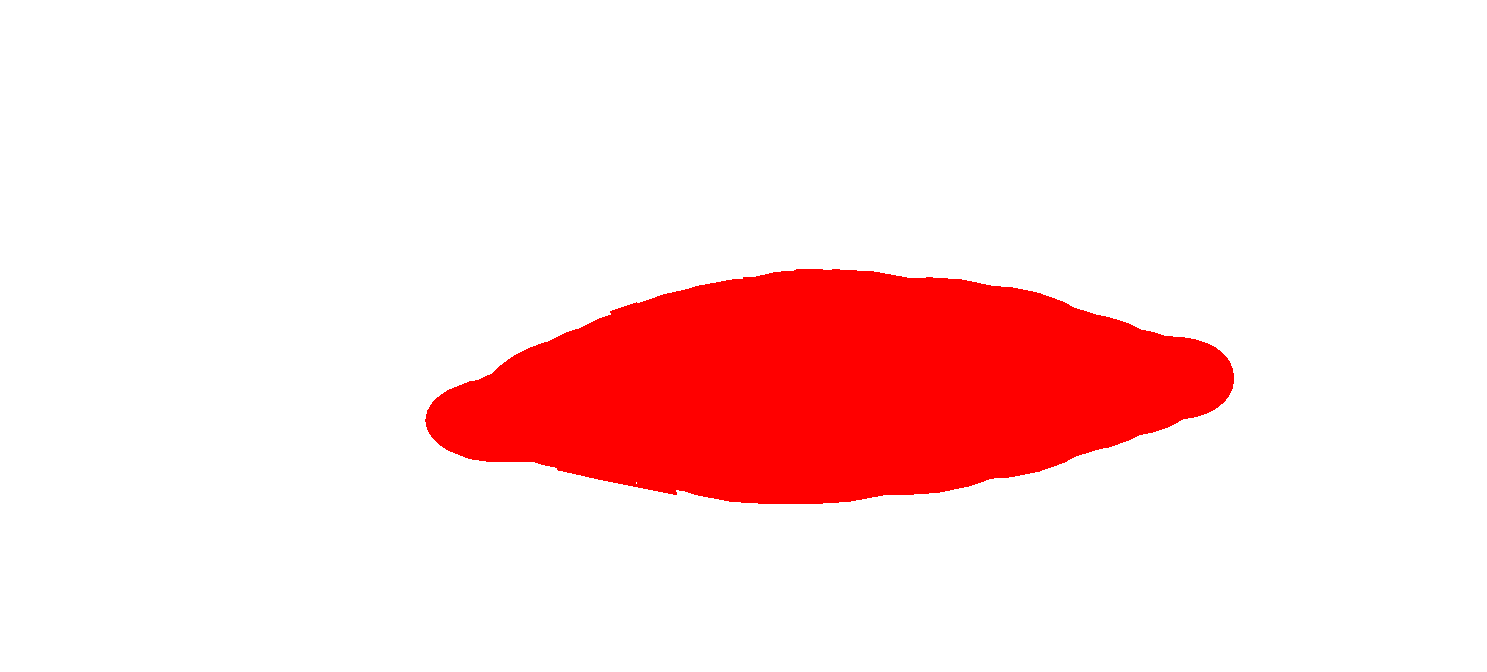If  and , find

Question 18:

If show that  exists for all real x, and find it.

It is known that,

Therefore, when x ≥ 0,

In this case,  and hence,

When x < 0,

In this case,  and hence,

Thus, for exists for all real x and is given by,

Question 19:

Using mathematical induction prove that  for all positive integers n.

For n = 1,

∴P(n) is true for n = 1

Let P(k) is true for some positive integer k.

That is,

It has to be proved that P(k + 1) is also true.

Thus, P(k + 1) is true whenever P (k) is true.

Therefore, by the principle of mathematical induction, the statement P(n) is true for every positive integer n.

Hence, proved.

Question 20:

Using the fact that sin (A + B) = sin A cos B + cos A sin B and the differentiation, obtain the sum formula for cosines.

Differentiating both sides with respect to x, we obtain

Question 21:

Does there exist a function which is continuos everywhere but not differentiable at exactly two points? Justify your answer ?

y=x           -∞<x≤1    2-x         1≤x≤∞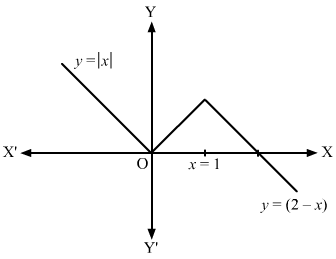It can be seen from the above graph that, the given function is continuos everywhere but not differentiable at exactly two points which are 0 and 1.

*Question 22:

Thus,

Question 23:

If show that

It is given that,

## Conclusion

NCERT solutions for Class 12 Math Chapter 5 “Continuity and Differentiability” are designed to provide a deeper understanding of the concepts of continuity and differentiability of functions. These concepts play an essential role in calculus and are fundamental to understand higher-level mathematics.
The chapter begins with the definition of continuity and its different types, such as pointwise continuity and uniform continuity. The chapter then proceeds to discuss differentiability and the relationship between continuity and differentiability.
NCERT solutions for Class 12 Math Chapter 5 provide detailed explanations of the concepts along with a variety of solved examples and exercises to reinforce the learning. Additionally, the solutions offer step-by-step guidance on how to approach different types of problems and help students to develop problem-solving skills.
Overall, NCERT solutions for Class 12 Math Chapter 5 are an essential resource for students who want to master the concepts of continuity and differentiability and develop their skills in calculus.

### Why do students prefer SWC’s NCERT Solutions for math class 12?

One of the top IIT JEE coaching institutes is Swastik Classes. Shobhit Bhaiya and Alok Bhaiya, pioneering mentors of IIT JEE Coaching Classes, started Swastik Classes in Anand Vihar. Over the last 15 years, they have educated and sent over 2000+ students to IITs and 5000+ students to different famous universities such as BITS, NITs, DTU, and NSIT. When it comes to coaching programmes for IIT JEE, Swastik Classes is the top IIT JEE Coaching in Delhi, favoured by students from all over India.

Swastik Classes’ teachers have a solid academic background, having graduated from IIT with honours, and have extensive expertise in moulding students’ careers.

The study process in Swastik courses is separated between pre-class and post-class work, which is one of the most significant aspects. They are precisely created to improve the student’s mental ability and comprehension.

## FAQS on NCERT Solutions for Class 12 Math Chapter 5

### What is continuity and differentiation?

One of the most essential subjects in mathematics is continuity and differentiability, which helps students grasp concepts like continuity at a point, continuity on an interval, derivative of functions, and many more. Continuity and Differentiability of functional parameters, on the other hand, are extremely difficult to achieve.

### What are the four relationships between differentiability and continuity?

If a function has a derivative, it is differentiable. A function’s derivative can be thought of as its slope. Continuous functions and differentiability have the following relationship: all differentiable functions are continuous, but not all continuous functions are differentiable.

### Is differentiation and differentiability same?

Differentiability relates to the presence of a derivative, whereas differentiation refers to the act of taking the derivative. As a result, we may state that any function can only be differentiated if it is differentiable.

### How is continuity and differentiability related?

The relationship between continuity and differentiability is that all differentiable functions are also continuous, but not all continuous functions are.

### Who discovered continuous function?

Bolzano discovered continuous function.# NCERT Solutions Class 12 Maths Chapters

• Chapter 1 Relations and Function
• Chapter 2 Inverse Trigonomtry
• Chapter 3 Matrices
• Chapter 4 Determinants
• Chapter 5 Continuity and Differentiability
• Chapter 6 Applications of Derivatives
• Chapter 7 Integrals
• Chapter 8 Application of Integrals
• Chapter 9 Differential Equations
• Chapter 10 Vector
• Chapter 11 Three Dimensional Geometry
• Chapter 12 Linear Programming
• Chapter 13 Probability